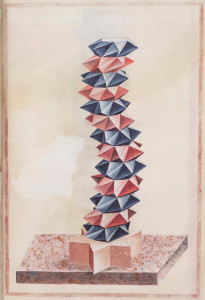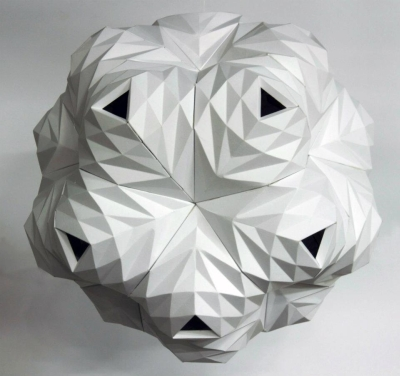# You're reading: Posts Tagged: polyhedra

### Radii of polyhedra

(At last month’s big MathsJam conference, we asked a few people who gave particularly interesting talks if they’d like to write something for the site. A surprising number said yes. First to arrive in the submissions pile was this piece by Tom Button.)

The formula for the surface area of a sphere, $A=4\pi r^{2}$, is the derivative of the formula for the volume of a sphere: $V=\frac{4}{3}\pi r^{3}$.

This result does not hold for a cube with side length $a$ if the surface area and volume are written in terms of $a$. However, if the surface area and volume are written in terms of half the side length, $r=\frac{a}{2}$, you get the surface area $A=24 r^{2}$, which is the derivative of the volume, $V=8 r^{3}$.

### Zero Waste by Nick Sayers

Get ready to smile!

[youtube url=http://www.youtube.com/watch?v=x7Xq4OX7beU]

### Knitted Spiky IcosahedronAs an avid knitter, and mathematician, the birth of a small human in my family inspired me to create a mathematical toy for the tiny person to enjoy while learning about shapes. With my favourite platonic solid being the icosahedron, it was the obvious choice for a knitted toy, and with stellation being all the rage, sticking a point on each face was the obvious next step, especially when it’s such a convenient thing for tiny inexperienced hands to grasp.

### Money Polyhedra by Kristi Malakoff### Perspectives of the regular solid

BibliOdyssey has posted some very old perspective drawings of polyhedra and other geometric shapes.### PRISMATICA by Kit Webster

[vimeo url=https://vimeo.com/37388088]

PRISMATICA by Kit Webster

### Paper light shades by Lazerian